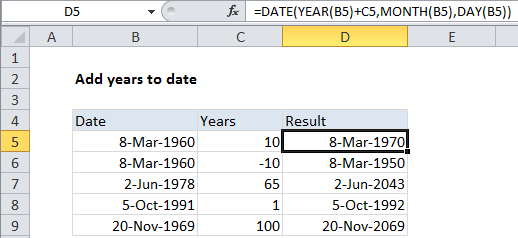## Excel Office

Excel How Tos, Tutorials, Tips & Tricks, Shortcuts

# Add years to date in Excel

If you need to add years to date in Excel then this tutorials is for you. See the example below .

To add a given number of years to a date, you can use a formula based on the DATE function, with help from the YEAR, MONTH, and DAY functions.

## Formula

`=DATE(YEAR(date)+years,MONTH(date),DAY(date))`Note: if you need to add an “even” multiple of 12 months to a date (i.e. 12, 24, 36, 48, etc) you can use a much simpler formula based on the EDATE function.

Also See:   Convert text date dd/mm/yy to mm/dd/yy in Excel

## Explanation

In the example shown, the formula in D5 is:

`=DATE(YEAR(B5)+C5,MONTH(B5),DAY(B5))`

### How this formula works

Working from the inside out, the YEAR, MONTH, and DAY functions extract those respective date components:

```=YEAR(B5) // 1960
=MONTH(B5) // 3
=DAY(B5) // 8```

At the outer level, the DATE function simply reassembles the component values back into a valid Excel date. To add years to the date, we just need to add the value in C5 to the year component prior to reassembly:

`=DATE(YEAR(B5)+C5,MONTH(B5),DAY(B5))`

The formula is then solved like this:

```=DATE(1960+10,3,8)
=DATE(1970,3,8)
=8-Mar-1970```
Also See:   Get fiscal quarter from date in Excel